# A Primer of the Parallel Constraint-Satisfaction Network Model of Decision Making

Version 9th April 2015

## Decision Situation

### Choice Options

Participants are asked to choose the more profitable stock in a sequence of $$z = \{1,\ldots,Z\}$$ pairwise comparisons between stocks.

Which stock is more profitable?

1. Trial: Stock 1 or stock 2
2. Trial: Stock 3 or stock 4

$$\vdots$$

Z. Trial: Stock $$(2 \times Z)-1$$ or stock $$2 \times Z$$

### Experts (Cues)

In each comparison, there are stock-market experts that either speak for or against a stock.

Experts differ in their ability to predict the better stock. The predictive accuracy of the experts is unknown to the participants.

### Example: Cue-pattern

Stock 1 Stock 2
Expert A + -
Expert B + -
Expert C - +

In the first comparison between stock 1 and 2, expert A and B speak for stock 1 and against stock 2 while expert C speaks against stock 1 and for stock 2.

## Model

The Structure of the Parallel-Constraint-Satisfaction Network Model of Decision Making (PCS-DM)

### Nodes: Elements in the Decision Task

Each element in the decision trial (experts and stocks) are represented as nodes in the network. Decision nodes (stocks) are displayed in the upper layer while cue nodes (experts) are displayed in the lower layer.

[The exemplary decision trial is displayed on the bottom.]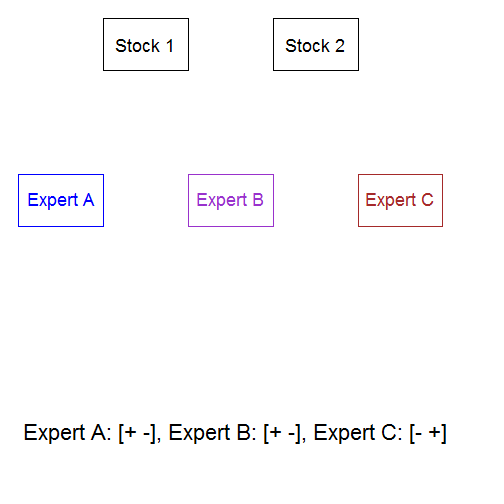### Weights: Cue-Pattern

Each expert is linked to each stock. The sign of the weights attached to the links represent the cue-pattern of the current decision trial.

Example

Expert A speaks for Stock 1 ($$+.01$$) and against Stock 2 ($$-.01$$).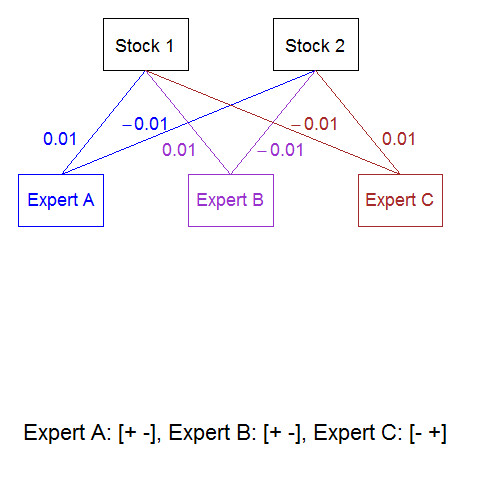### Weight: Forced Choice

Since participants are asked to decide which of two stocks is more profitable (i.e., forced choice), stocks are linked with a high negative link.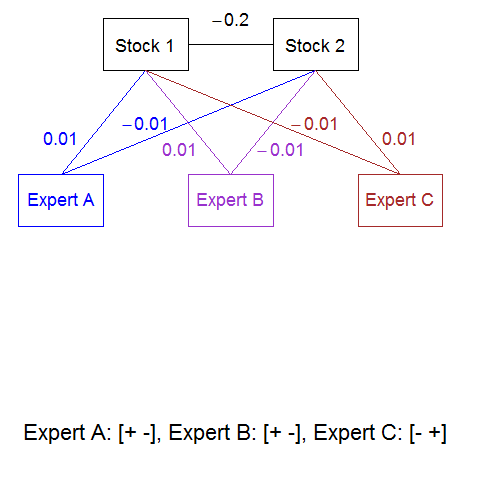### Weights: Subjective Validities

A source node activates each of the experts in the decision process. Cue-validities are represented as weights attached to the links. Weights can change through learning.

[In the example, the decision maker/network thinks that Expert $$A$$ is more predictive than $$B$$ and Expert $$B$$ is more predictive than $$C$$.]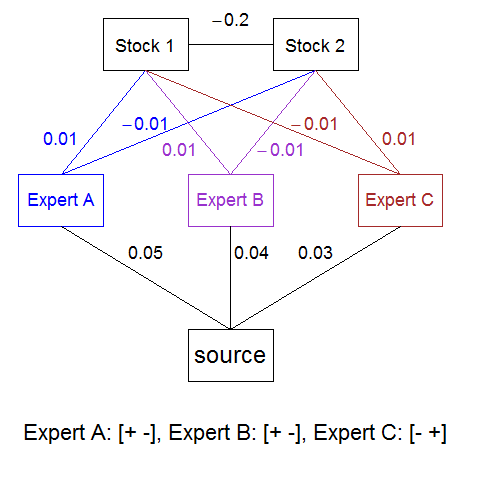## Decisions

Modelling the Decision Process in PCS-DM

### Iteration 1

The decision process is modelled as activation spreading from the source node into the network over iterations $$i = \{1,2,\ldots,I\}$$.

In the first iteration, the source node receives an activation of 1.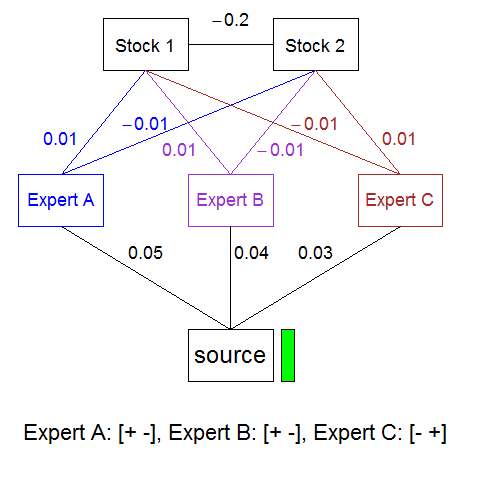### Iteration 2

Activation spreads from the source node to the cue nodes relative to the subjective validities of the experts.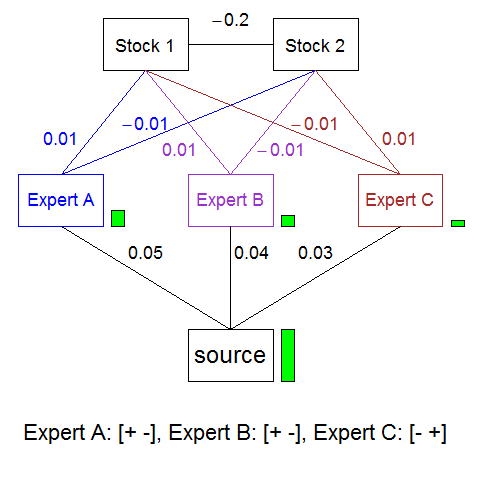### Iteration 3

Activation continues to spread from the source node to the cue nodes. Additionally positive or negative activation flows from the cue nodes to the option nodes according to the cue-pattern.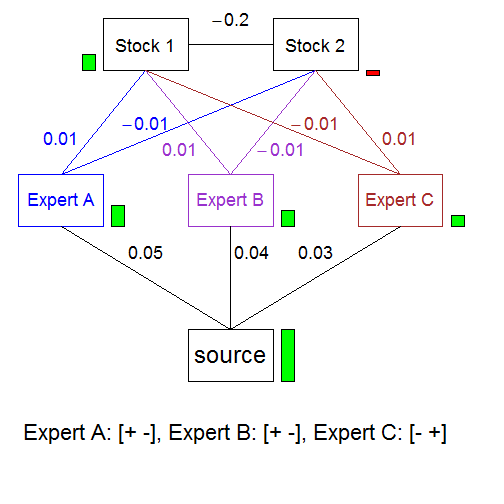### Iteration 4

Activation spreads forwards but also backwards from the option nodes to the cue nodes resulting in a negative activation of expert 3.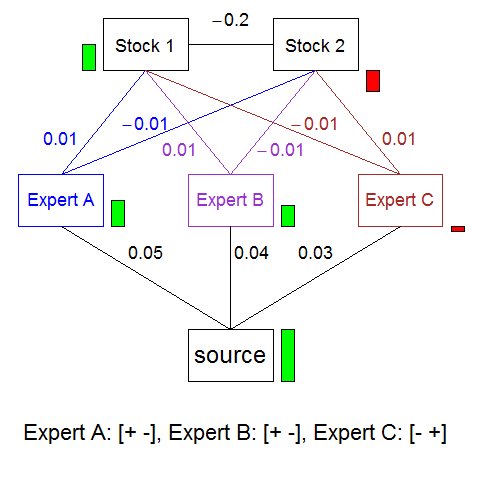### Iteration I

At the final iteration I, activations in the network do not change anymore. The predicted choice can be read from the activations of option nodes.

PCS-DM predicts that Stock 1 is chosen.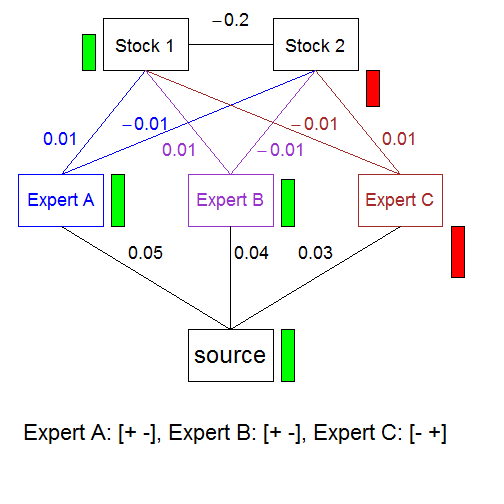### Dependent Measures

The model allows deriving predictions for several measures:

• Participants choose the option with the higher activation.
• Participants' decision time increases with an increasing number of iterations $$I$$ needed for reaching a stable solution.
• Participants' confidence in a decision increases with increasing difference in activations for option-nodes (stocks).

### Demo

An animation of the decision process for the exemplary cue-pattern can be found here.

In the left display, the current activation of nodes is shown; in the right display, activations are plotted over iterations.

Note that activations of cues are driven by the source node until cues are influenced by backward activation around iteration #36 (i.e., activation for expert 3 slightly decreases).

### Overview

Node activations in each iteration are updated in three steps:

1. Nodes receive input from other nodes. The input is calculated as the weighted sum of activations of nodes connected to the node.
2. Input is transformed into an activation to let node-activations vary between $$-1$$ and $$+1$$.
3. The overall coherence of the PCS-network is calculated. If the extent of coherence does not change significantly over iterations, iterative updating is stopped.

### Node Input

The input of a node p at iteration i is calculated as the weighted sum of all nodes linked to the node:

$input_{node_p,i} = \sum_{q = 1, q \neq p}^{Q} w_{node_q-node_p} \times a_{node_{q},i}.$

Example

In case Stock 1 has an activation of $$.3$$ and Stock 2 an activation of $$-.3$$ at iteratrion # 30, the input for Expert A is:

$input_{\text{Expert A},i = 30} = 2 \times .003 + 1 \times .05 = .056.$

### Tranforming Input into Activation

The input of a node is transformed into an activation:

$a_{node_{i+1}} = a_{node_{i}} \times (1-decay) + input_{node_{p,i}} \times x$

with

$x = \left\{ \begin{array}{lr} a_{node_p,i} + 1 & \text{if } input_{node_p,i} < 0\\ 1 - a_{node_p,i} & \text{if } input_{node_p,i}\geq 0 \end{array} \right.$

Example

In case Expert A had an activation of .2 at iteration # 30 and $$decay$$ is .1, the input results in an activation of:

$a_{\text{Expert A},i = 31} = .2 \times .9 + .056 \times (1 -.2) = .22.$

### Coherence (Negative Energy)

The coherence (or negative energy) measures how well the activations of the nodes at iteration $$i$$ in the network fit to each other:

$energy_{i} = - \sum_{p = 1}^{P = N} \sum_{q = 1, q \neq p}^{Q = N} w_{node_p-node_q,i} \times a_{node_p,i}\times a_{node_q,i}$

Low values of energy indicate a coherent network.

Example

A network is more (less) coherent when two positive activations are connected by a positive (negative) link to each other.

## Learning

Updating Cue-Validities in PCS-DM Through Learning

### Case 1: Incorrect Choice

The network chooses Stock 1 but is informed that Stock 2 is more profitable: PCS should have produced the activations for Stocks indicated by the blue bars: A highly negative activation for Stock 1 and a highly positive activation for Stock 2.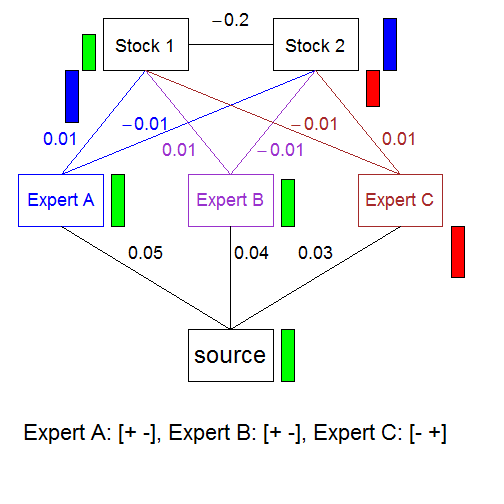### Updating Validity Weights

A network-model that reduces the difference between the observed (green/red bars) and desired (blue bars) activations for stocks consists of a lower validity weight for Expert A and B and a higher weight for Expert C.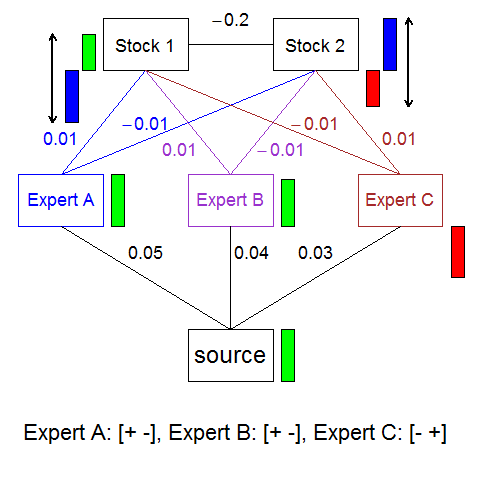### Case 2: Correct Choice

A correct choice also leads to a change in valdity weights. The difference between desired and observed activations is reduced by increasing the weights for Expert A and Expert B and decreasing the weight for Expert C.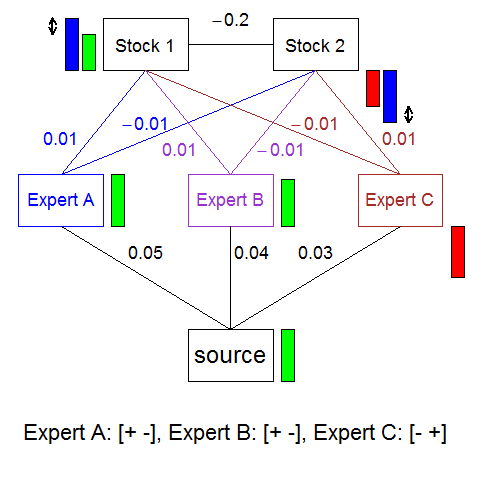### Overview

Updating of validity weights consists of three steps:

1. Validity weights are changed in proportion to the difference between desired and observed activations for stocks.
2. Changes in weights are transformed to let weights vary between $$-1$$ and $$+1$$.
3. Transformed changes of validity weights are added to the validity weights.

### Delta Rule

Change in validity weights is determined by the difference between desired and observed activations of stocks and an individual learning rate $$\lambda$$:

$\Delta w_{cue_q,t}= \lambda \times \sum_{p=1}^{P}[(d_{a_{opt_{p},I,t}}-a_{opt_{p},I,t}) \times w_{cue_q-opt_p,t}]$

Example

In case Stock 1 had an activation of $$.6$$ and Stock 2 had an activation of $$-.6$$ and the desired activation is $$-1$$ and $$1$$ for stocks, and $$\lambda = 1$$, the validity weight of Expert 1 is updated by:

$\Delta w_{\text{Expert A},t} = -1.6 \times .01 \times 2 = -.032.$

### Transformation function

To let validity weights vary between $$-1$$ and $$+1$$, changes in validity weights are transformed:

$f(\Delta w_{cue_p,t}) = \Delta w_{cue_p,t} \times \left\{ \begin{array}{lr} (1 - w_{cue_p,t}) & \text{if } w_{cue_p,t} \geq 0\\ (1 + w_{cue_p,t}) & \text{if } w_{cue_p,t} < 0 \end{array} \right.$

Example

The transformed change in the validity weight of Expert 1 is:

$f(\Delta w_{\text{Expert A},t}) = -.032 \times (1 - .05) = -.03.$

### Updating Validity Weights

The validity weights are updated according to:

$w_{cue_p,t+1}=w_{cue_p,t}+f(\Delta w_{cue_p,t}).$

Transformed changes in validity weights are added to the validity weights.

Example

The validity weight of Expert 1 is adjusted to:

$w_{\text{Expert A},t+1} = .05 - .03 = .02.$